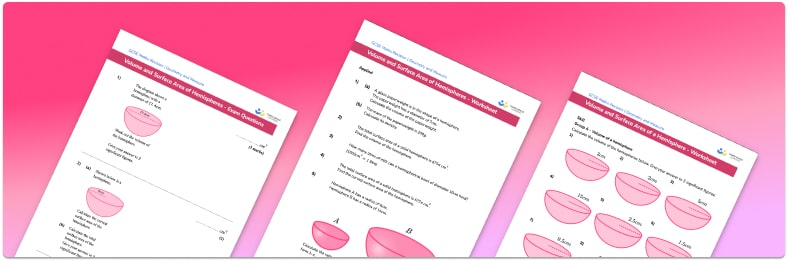# Volume And Surface Area Of A Hemisphere WorksheetHelp your students prepare for their Maths GCSE with this free volume and surface area of a hemisphere worksheet of 30+ questions and answers

• Section 1 of the volume and surface area of a hemisphere worksheet contains 20+ skills-based volume and surface area of a hemisphere questions, in 3 groups to support differentiation
• Section 2 contains 3 applied volume and surface area of a hemisphere questions with a mix of worded problems and deeper problem solving questions
• Section 3 contains 3 foundation and higher level GCSE exam style volume and surface area of a hemisphere questions
• Answers and a mark scheme for all volume and surface area of a hemisphere questions are provided
• Questions follow variation theory with plenty of opportunities for students to work independently at their own level
• All questions created by fully qualified expert secondary maths teachers
• Suitable for GCSE maths revision for AQA, OCR and Edexcel exam boards

• This field is for validation purposes and should be left unchanged.

You can unsubscribe at any time (each email we send will contain an easy way to unsubscribe). To find out more about how we use your data, see our privacy policy.

### Volume and surface area of a hemisphere at a glance

A hemisphere is half of a sphere. The volume of a hemisphere is the amount of space within the hemisphere and the surface area of a hemisphere is the total area of the faces of the hemisphere.

To find the volume of a hemisphere, we find the volume of the whole sphere using the formula: V = frac{4}{3}πr^{3}, where r is the radius of the sphere. (The radius of a sphere is the distance from the centre of the sphere to the edge of the sphere and the diameter of a sphere is the distance from one side of the sphere to the other, through the centre of the sphere). We can half the volume of the sphere to find the volume of the hemisphere.

To find the surface area of a hemisphere, we find the surface area of the whole sphere using the formula SA = 4r^{2}. We can then half the surface area of the sphere to find the curved surface area of the hemisphere. To find the total surface area of a hemisphere, we then need to add the area of the circular base, found using the formula A = πr^{2}.

Sometimes we are asked to give an exact answer in terms of π. Other times we might be asked to round the answer to a given number of decimal places, to the nearest integer or to a given number of significant figures.

We usually use metric units for measurement and volume is measured in cubic units such as cm^3 and surface area in square units such as cm^2.

Looking forward, students can then progress to additional 3D shapes worksheets and other geometry worksheets, for example an angles in polygons worksheet or volume and surface area of spheres worksheet.For more teaching and learning support on Geometry our GCSE maths lessons provide step by step support for all GCSE maths concepts.

## Do you have KS4 students who need more focused attention to succeed at GCSE?There will be students in your class who require individual attention to help them succeed in their maths GCSEs. In a class of 30, it’s not always easy to provide.

Help your students feel confident with exam-style questions and the strategies they’ll need to answer them correctly with our dedicated GCSE maths revision programme.

Lessons are selected to provide support where each student needs it most, and specially-trained GCSE maths tutors adapt the pitch and pace of each lesson. This ensures a personalised revision programme that raises grades and boosts confidence.

Find out more